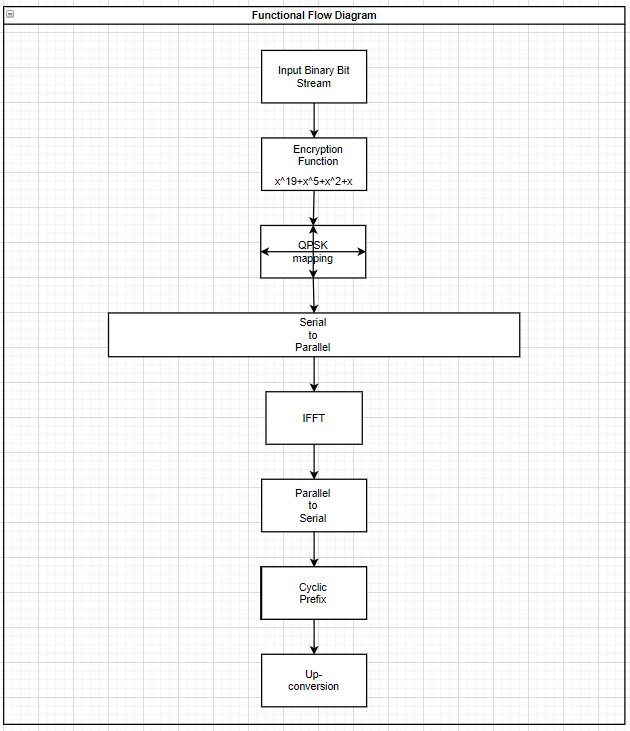## Assistance with Communication System Homework Involving LTE

The LTE system is supposed to work like this. First, we took a bitstream of 2,097,152 bits, and we encrypted it using an MSRG with the polynomial x19‬ + x5 + x2 + x. Afterward, we mapped every 2 bits of the encrypted bitstream to the QPSK constellation diagram with respect to the standard TS 36.211 V8.9.0 Section 7.1.2 (image 1). Next, in this communication system homework solution, we transformed the symbol from being in series to parallel. We didn’t need to account for multiple users sharing the bandwidth because we only used one bitstream data for this project. With this parallel symbol, we can take the IFFT for the 1024 subcarriers. Then after we get the symbol, we add the last part of the signal to the beginning, giving us a cyclic prefix. Finally, we upconvert the frequency of each of the symbols to that of a subcarrier.

## Transferring Data as a Part of Assistance with Communication System Assignment

What is an LTE system? LTE stands for Long-Term Evolution and is currently one of the biggest methods in transferring data to an internet protocol system. Rather than using small amounts of data for transfer, LTE mobilizes large packets of data at a time. For instance, our LTE implementation requires us to transfer over 2 million bits. Moving the data around and encrypting it all in an orderly fashion. For our communication system assignment help service, we used Matlab to simulate an LTE system and run a given test sequence to attain a specific output. Below is a flow diagram representing each of the steps.

Functional Flow Diagram of the System:1. Input binary bitstream
2. Apply encryption function
3. Mapping results to QPSK symbols
4. Convert serial to parallel
5. Map complex symbols to the input of IFFT
6. Perform IFFT
7. Convert for parallel to serial